# Theoretical and experimental analysis of a new compressible flow small power turbine prototype

Theoretical and experimental analysis of a new compressible flow small power turbine prototype

Silvio Barbarelli*Gaetano Florio Nino Michele Scornaienchi

Department of Mechanical, Energy and Management Engineering, University of Calabria

Corresponding Author Email:
silvio.barbarelli@unical.it
Page:
S391-S398
|
DOI:
https://doi.org/10.18280/ijht.35Sp0153
|
Accepted:
|
Published:
30 September 2017
| Citation

OPEN ACCESS

Abstract:

An innovative compressible-flow small turbine prototype with rotating channel and with 3 optimized deflectors is presented. The prototype is able to recover energies usually lost deriving for example from exhaust gases or waste steams characterized by low pressures and small flow rates. The non-typical turbine configuration allows reaching good performances in different operating conditions. A 70-kW maximum brake power, pressured-air test rig has been set up for gathering global measurements such as pressure, temperature, flow rate, torque and rotational speed. First results show that the overall efficiency of the turbine prototype is comparable with the other small-power configurations, but its rotational speed is significantly reduced. In this paper, the authors provide experimental measurements of the prototype together with a numerical model able to predict its performances.

Keywords:

compressible flow, tangential flow small turbine, rotary channel, test rig

1. Introduction

The demand of energy is today hugely increased, despite of the reduction of traditional sources. In this scenario is fundamental to recognize and utilize renewable and sustainable energy technologies or optimize existing processes [1-2]. Particularly, to exploit energy usually not utilized, including waste energy and possibilities offered for example by lamination valves found in various industrial processes, can be used small turbines. These micro turbines [3-4] can be merged with existing systems, reducing so the costs through electricity production or a direct drive of other devices. Some applications involve incineration plants, sugar factories, heat exchange stations, heating plants, food industries, solar power plants , biomass power plants .

Z. Shao et al  propose an interesting application of energy recovery of exhaust gases by means of a micro-Pelton turbine.

In this field of research, the authors have developed several models of small tangential flow turbine prototypes, having a rotating channel fitted with 3 or 5 deflectors able to recover energy from exhaust gases or waste steams characterized by low pressures, under 10 bars, and small flow rates, under 200 g/s [8-9]. These prototypes have been installed and tested on a 70-kW maximum power test rig, working with both air and steam, equipped with suitable instruments and with an electronic data acquisition system .

Experimental campaigns, recording flow, pressure, temperature, torque and rotational speed, have been carried out with the aim to verify the working principle. These measurements clearly demonstrated that the prototype achieves acceptable performances, also in off design conditions. In this paper, the authors focus both on theoretical and experimental aspects of the prototype with three deflectors, by implementing a numerical model able to predict the overall performances of the prototype itself.

2. Construction Criteria of the Turbine Prototype

## 1.pngFigure 1. Pattern of the three deflectors prototype

When a small turbine is designed with very low inflow capacities, the constructive criterions have to take into account a low partial admission annulus degree.  That implies blades losses very high, which decrease the overall performances of the machine to unacceptable values.

In this case, one or more nozzles can supply the necessary inflow tangentially to the machine, which assumes so a micro Pelton configuration . The innovative prototype presented in this paper, characterized by its small size and constructive simpleness, was made with a rotary disk of 29 mm width and a 175-mm diameter. The height and width of the flow passage section are respectively 11 mm and 7 mm, while the angle of the deflector axis and the tangential direction is 20°.

As shown in Fig. 1, it is a tangential flow turbine characterized by a rotating channel with deflector ducts inside, fed by air through a converging nozzle, whose opening is varied by a suitable moving spear (Fig. 1) inserted in the stator.

Indeed, by means of a continuous screw, it is possible to vary the opening of the nozzle from zero to the cross-section area of the rotating channel. The percentage changes are represented by the spear opening coefficient, defined as it follows:

$Kp=\frac{\text{nozzle area}}{\text{channel area}}$  (1)

3. Test Rig

The test rig, illustrated in Fig. 2, allows global quantity measurements, such as power, rotational speed number, torque, flow rate, pressure and temperature. It is equipped with a data acquisition system enabling the control, the management and the recording of automated measurements. The essential components of the test rig are:

- screw action volumetric compressor;

- dynamometric brake fitted with a regulation and control system;

- electronic flow rate measurement device;

- temperature measurement devices (thermoresistors and thermocouples);

-mechanical and electronic pressure measurement devices;

-data acquisition board;

-computer.

The compressor is an oil-cooled screw-action rotary volumetric type. It can supply an air flow rate of 13000 lt/min, while its pressure is 8 bars. (rated conditions).

The dynamometric brake on which the turbine dissipates its power is an eddy-current type with a water-cooling system connected to the local water supply. The brake is fitted with a control module able to impose the number of revolutions per minute. It is able to adsorbe100 kW maximum power at a rotational speed of 15000 rpm. Moreover, the brake is equipped with a piezometric load cell, which records the load torque, and with a digital tachymeter, which measures the rotational speed of the turbine.

The flow rate device is composed of a short sharp edge orifice ISA 1932 and an electronic differential manometer.

The temperature measurement devices are 3 digital thermocouples located near the sharp edge orifice, and at the turbine inlet and outlet.

There are 3 mechanical Bourdon-tube gages: two placed on the cooling pipes of the dynamometric brake and one at the outlet of the turbine. There is also an electronic pressure gage installed on the turbine inlet pipe and 2 piezometric sensor for the pressure measurements inside the rotary channel.

Signals from the various electronic measuring instruments and from the control module of the dynamometric brake (see Fig. 2), are gathered by a terminal screw, and sent, by an insulated cable, to the data acquisition board. A suitable software, developed in LabView environment, provides the monitoring, the management and the recording of the data coming from the various measuring instruments.

## 2.png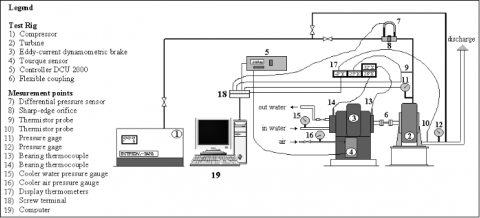Figure 2. Test rig

4. Global Measurements

The global measurements were carried out by changing the spear opening coefficient Kp (from 0.2 to 1), the inlet pressure po (from 3.5 to 6 bar) and the rotation regime (from 0 to 12000 rpm). Below the minimum values indicated, the turbine cannot provide useful power for the considered rotation regime range. The upper limit values are imposed by the power of the compressor. The range of values above indicated represents anyway the working field of the machine.

Figures from 3 to 6 plot the efficiency curves as a function of the velocity ratio u/co, for different values of the spear opening (Kp) and of the feeding pressure (po). The curves show the typical parabolic trend of a simple action stage: the turbine reaches the highest efficiencies for values of the parameter u/co falling in the range 0.25¸0.3. In particular the maximum achieved efficiency is 42.5%, obtained for po = 3.5 bar, Kp = 0.6 e u/co = 0.27. In the next section, the punctual measurements of the pressure in the rotary channel will be illustrated.

## 3.png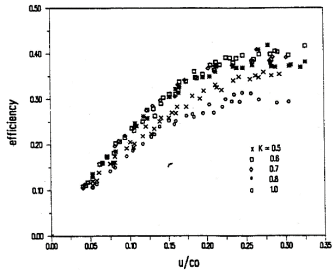Figure 3. Efficiency curves – po = 3.5 bar

## 4.png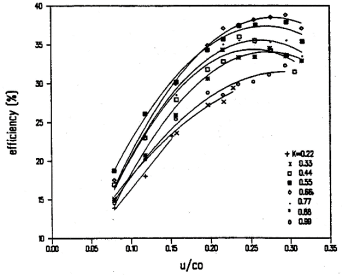Figure 4. Efficiency curves – po = 4 bar

## 5.png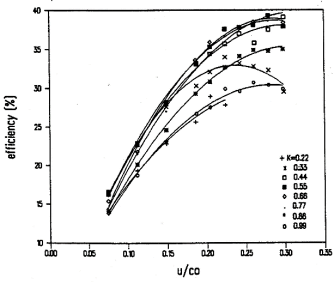Figure 5. Efficiency curves – po = 5 bar

## 6.png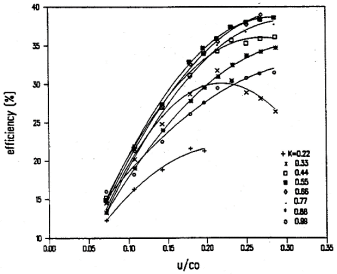Figure 6. Efficiency curves – po = 6 bar

5. Puntual Pressure Measurements

Preliminary considerations on how the flow evolves inside the rotary channel are necessary before to analyze the pressure measurements. The different flow ways depend on the position of a generic deflector t respect to the feeding nozzle (see Fig. 7). Taking into account that two consecutive deflectors c and t define a working sector of the turbine, highlighted in gray in Fig. 7, three phases are detected, described as it follows.

Transition phase: the jet, for a small rotation arc, invests two sectors (see Fig. 7a). The inert fluid, contained in the sector highlighted in gray, is mixed with the pressurized fluid coming from the nozzle, creating so turbulences and pressure oscillations. This phase ends when the deflector t of the gray sector is frontal to the main jet (see Fig. 7b).

Filling phase: the flow feeds only the gray sector Fig. 7c. In this phase, the pressure inside the channel reaches an intermediate level between the choking and atmospheric pressure, and the outflow through the deflector is supersonic.

Discharge phase: the tail deflector c of the sector under consideration is in front of the main jet (see Fig. 7d). In this case, the sector is not connected with the inlet flow. After a certain angle of rotation (see Fig. 7e), the flow through the deflector t ends and the sector remains filled by inert fluid. The discharge phase ends when the head deflector t meets the nozzle area (see Fig. 7f) and so the transition phase starts again.

These three phases are cyclically repeated: in a complete rotation each sector, for about 1/3 of the time is filled by the nozzle, for about 2/3 of the time it discharges the excess fluid by empting itself and for a relatively short time it is in the transition phase.

## 7.png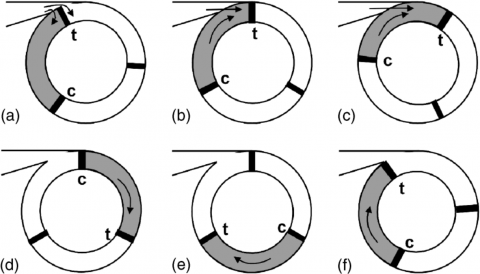Figure 7. Sequence of the flowing phases

With the aim to record the pressure oscillations  within the sectors, two piezoelectric pressure sensors have been inserted in the positions highlighted in figure 8.

## 8.pngFigure 8. Piezoelectric pressure sensors position

The next figures, from 9 to 14, show the pressure oscillations recorded in the sectors by the two pressure pickups, for three operating conditions of the parameters Kp, po and rpm.

## 9.png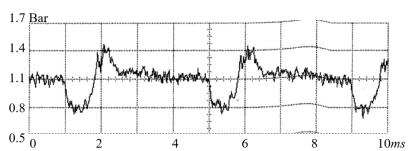Figure 9. Pressure signal recorded by sensor 1 po = 3 Bar, Kp = 0.66, rpm = 5000

## 10.png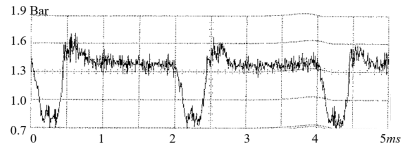Figure 10. Pressure signal recorded by sensor 1 po = 4 Bar, Kp = 0.66, rpm = 10000

## 11.png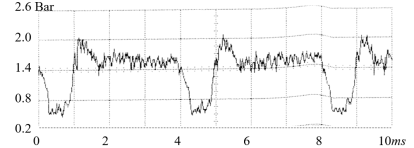Figure 11. Pressure signal recorded by sensor 1 Po = 5 Bar, Kp = 1, rpm = 5000

## 12.pngFigure 12. Pressure signal recorded by sensor 2 po = 3 Bar, Kp = 0.66, rpm = 5000

## 13.png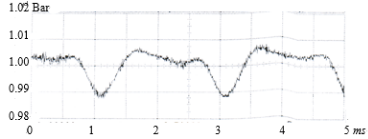Figure 13. Pressure signal recorded by sensor 2 po = 4 Bar, Kp = 0.66, rpm = 10000

## 14.pngFigure 14. Pressure signal recorded by sensor 2 po = 5 Bar, Kp = 1, rpm = 5000

The measurements of sensor 1 show clearly that the pressure of each sector during the transition phase almost arises to the choking value. Successively, during the filling phase, reaches values between the choking value and the atmospheric value. Finally, during the empting phase decreases to values under that atmospheric.

The measurements of sensor 2 show instead weak oscillations around the atmospheric value.

These measurements have been utilized to develop a numerical model able to predict the performances of the turbine prototype, which is introduced in the next section.

6. Numerical Model

After identified the three different flow phases, and the pressure oscillations in the rotary channel, it is possible to write the equations of the fluid motion within the turbine and thus develop the theoretical expressions related to the performances of the machine. The dependence between the flow parameters and the deflector position of each individual sector is highlighted by the angle q, as shown in Fig. 15.

## 15.png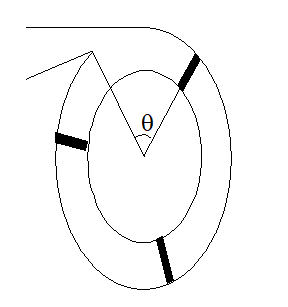Figure 15. Flow angle

The work per unit mass is given by:

$L(\theta )=(1+\cos \beta )(c(\theta )-u)\cdot u$   (2)

The power is instead given by:

$P(\theta )=\overset{.}{\mathop{m}}\,(\theta )\cdot L(\theta )$      (3)

The total work done during the passage of a single sector is equal to:

${{L}_{tot}}=\int_{\sec t}^{{}}{P(\theta )\cdot \frac{d\theta }{\omega }}$ (4)

By considering that the turbine has z sectors (in the present case, z = 3) the power is equal to:

P= z Ltot n (5)

Instead, referring to spouting velocity co, the efficiency is given by:

$\eta = \frac{z\text{ }{{L}_{tot }}\text{ }n\text{ }}{Kp{{\overset{.}{\mathop{m}}\,}_{1}}\frac{c_{o}^{2}}{2}}$       (6)

The velocity variation c(q) is calculated with respected to the three flow phases before described.

6.1 Transition phase

In this phase, it is allowed to suppose a choked flow: all the thermo-fluid dynamic parameters are calculated by the traditional formulas related to the choking conditions . Particularly, the velocity is determined by the following equation:

${{c}_{1}}=\sqrt{kR{{T}_{1}}}=\sqrt{\frac{2k}{k+1}RTo}$    (7)

## 16.pngFigure 16.  Passage area during the transition phase

Referring to figure 16, the passage area is calculable as:

${{g}_{1}}(\theta )=2{{r}^{*}}b\sin \frac{2\theta _{p}^{*}-\theta }{2}\sin \frac{\theta }{2}$     (8)

6.2 Filling phase

With the aim to calculate the flow parameters a control volume Vc has been identified near to the main jet illustrated in Fig. 17.

## 17.png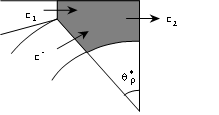Figure 17.  Control volume

The continuity and momentum equations can be applied taking into account the following hypothesis:

- one dimensional flow;

- no friction;

- no vortices;

- no leakages;

These equations are written referring to the control volume Vc, as it follows:

$\int\limits_{Vc}{\rho (\overset{\to }{\mathop{c}}\,}\cdot \overset{\to }{\mathop{n}}\,)dA\text{ = }0$    (9)

$F\text{ = -}\int\limits_{Vc}{\rho \overset{\to }{\mathop{c}}\,}(\overset{\to }{\mathop{c}}\,\cdot \overset{\to }{\mathop{n}}\,)dA$   (10)

After some passages, equations 9 and 10 become:

$Kp{{\rho }_{1}}{{c}_{1}}\text{ = }{{\rho }_{2}}({{c}_{2}}-{{c}^{*}})$     (11)

$Kp({{p}_{1}}-{{p}_{2}})\text{ = }{{\rho }_{2}}c_{2}^{2}-Kp{{\rho }_{1}}c_{1}^{2}-{{\rho }_{2}}{{c}^{*2}}\cos \theta _{p}^{*}$    (12)

By solving the previous system, the following expression for the velocity c2 is obtained:

${{c}_{2}} = \frac{-A+\sqrt{{{A}^{2}}\cos \theta _{p}^{*}\text{ }+\text{ A}B\left( \frac{{{p}_{1}}-{{p}_{2}}+{{\rho }_{1}}c_{1}^{2}}{{{\rho }_{1}}{{c}_{1}}} \right)\text{ }}}{B} +A$    (13)

where:

$A=Kp\text{ }{{c}_{1}}{{\left( \frac{{{p}_{1}}}{{{p}_{2}}} \right)}^{\frac{1}{k}}}$     (14)

$A=Kp\text{ }{{c}_{1}}{{\left( \frac{{{p}_{1}}}{{{p}_{2}}} \right)}^{\frac{1}{k}}}$      (15)

The only value in eq. 13 not available from theoretical or geometrical data is the channel pressure p2. For covering this data the authors have collected suitable experimental measurements and subsequently interpolated them with the total pressure po, with the flow rate coefficient Kp and with the rotational speed n, obtaining so the following expression, having a R squared value of 0.96:

${{p}_{2}}=0.2905\text{ }{{\text{p}}_{\text{o}}}+0.9652\text{ }Kp+1.2445x{{10}^{-5}}\cdot n\text{-0}\text{.5819}$     (16)

6.3 Discharge phase

During this phase, the velocity is supposed to change linearly from the value c2 (see eq. 13) to the value of inert drag u. The empting angle qempt can be calculated by considering that in absence of feeding, the integral of the flow rate outgoing from the sector is equal to the excess mass, i.e:

$\int_{{{\theta }_{\text{1}}}}^{{{\theta }_{\text{res}}}}{\rho (\theta )}\cdot [c(\theta )-u]\cdot Ac\cdot \frac{d\theta }{\omega }=V\left( {{\rho }_{2}}-{{\rho }_{res}} \right)$     (17)

Taking into account that after the empting, the sector is filled by inert fluid simply dragged at the peripheral velocity u (residual condition), the empting angle is given by:

${{\theta }_{empt}}={{\theta }_{res}}-{{\theta }_{1}}=\frac{12u\pi ({{\rho }_{2}}-{{\rho }_{res}})}{z\text{ }({{c}_{2}}-u)(2{{\rho }_{2}}+{{\rho }_{res}})}$      (18)

Finally, the equations 5 and 6 related to the calculation of the power and of the efficiency, taking into account eqs. 7, 8, 13 and 18, become:

$P=z\text{ }(1+\cos \beta ){{A}_{c}}\cdot \left[ C\text{ }{{({{c}_{1}}-u)}^{2}}u+(D+E){{({{c}_{2}}-u)}^{2}}u \right]$     (19)

$\eta \text{ }=\text{ }\frac{2\text{ }z\text{ }(1+\cos \beta ){{c}_{o}}}{Kp{{\rho }_{1}}{{c}_{1}}}\left[ \text{C }{{\left( \frac{{{c}_{1}}}{{{c}_{o}}}-\frac{u}{{{c}_{o}}} \right)}^{2}}\frac{u}{{{c}_{o}}} \right.+(\left. D+E){{\left( \frac{{{c}_{2}}}{{{c}_{o}}}-\frac{u}{{{c}_{o}}} \right)}^{2}}\frac{u}{{{c}_{o}}} \right]$    (20)

where:

$C=\frac{Kp\text{ }{{\rho }_{1}}}{\pi (1-\cos \theta _{p}^{*})}\left( \frac{sen\theta _{p}^{*}}{2}-\frac{\theta _{p}^{*}\cos \theta _{p}^{*}}{2} \right)$     (21)

$D=\frac{{{\rho }_{2}}}{z}$     (22)

$E=\frac{{{\theta }_{empt}}}{12\pi }\left( 3{{\rho }_{2}}+{{\rho }_{res}} \right)$     (23)

7. Losses Calculation

The numerical model is completed with the computation of the fluid dynamic losses, whose main contributions derive from:

- friction losses;

- incidence losses;

- disc losses;

- leakage losses.

The friction losses are calculated by reducing, through a suitable coefficient j, the absolute flow velocity c1 in the nozzle, and, through a suitable coefficient y, the relative velocity c-u in the various sections of the turbine rotor.

The incidence losses are calculated by considering that, during the transition phase (see Fig. 18), the component of flowing velocity c1 useful to produce work is:

${{c}_{1}}\cos {{\theta }_{inc}}={{c}_{1}}\cos (\theta _{p}^{*}-\theta )$    (24)

The power disc losses have been calculated by means of the following expression:

$\Delta {{P}_{fr}}={{K}_{fr}}\rho D_{t}^{2}{{u}^{3}}$     (25)

where the coefficient Kfr has been experimentally determined as 3.496×10-3.

Finally, the leakage loss can be calculated by supposing the pressure drop between the channel and the casing to be low. In this case, the flow through clearances can be considered incompressible and then the following formula can be applied :

${{\overset{.}{\mathop{m}}\,}_{lk}}=\alpha {{A}_{lk}}\sqrt{{{\rho }_{2}}\frac{p_{2}^{2}-p_{atm}^{2}}{{{p}_{2}}}}$    (26)

## 18.png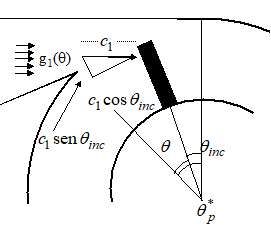Figure 18. Incidence effect

8. Comparison between Experimental and Numerical Data

In the next figures, from Fig. 19 to Fig. 21, a comparison between experimental data and theoretical curves is shown by changing the velocity ratio u/co, for different operating conditions of total pressure po and of flow rate coefficient Kp. The theoretical curves of efficiency agree with experimental data in all three cases: the highest values of the efficiency are always obtained for the velocity ratio u/co around 0.25. The maximum efficiency highlighted in these figures is about 40%, obtained for a total pressure po of 3 Bar, for a flow coefficient Kp of 0.5 and for a u/co velocity ratio u/co of 0.24.

## 19.png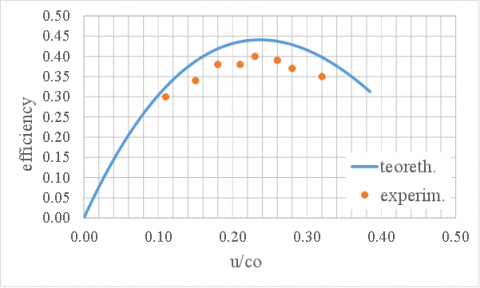Figure 19. Comparison between theoretical efficiency curve and experimental data for po = 3 Bar and Kp = 0.5

## 20.pngFigure 20. Comparison between theoretical efficiency curve and experimental data for po = 4 Bar and Kp = 0.66

## 21.png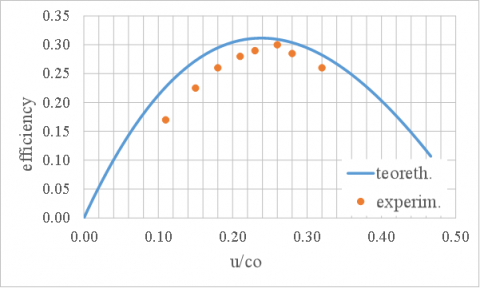Figure 21. Comparison between theoretical efficiency curve and experimental data for po = 5 Bar and Kp = 1

With the increasing of the flow coefficient Kp and of the feeding pressure po a worsening of the performances is observed: this phenomenon is mainly due to the admission area geometry and to the increasing of the channel pressure p2 (see eq. 16). In fact, the convergent-divergent nozzle configuration, which guarantees the high speed of the flow in the rotary channel (see Figs. 16, 17, 18), finds less and less ideal discharge conditions. This mean that shock waves are formed in the rotary channel by reducing so the speed flow velocity to lower values and consequently by worsening the overall performances.

This issue can be resolved in the next prototypes design, taking into account a better arrangement of the moving spear and of the rotary channel (see Fig. 1).

9. Conclusions

In this paper, a new low power gas turbine prototype has been presented, able to recover energy usually lost, deriving for example from gas exhaust or from waste streams, characterized by small flow rates and small pressure drops. The best performances obtained at relatively low rotation velocities represent an undoubted advantage of this prototype with respect to other machines of the same type.

Global measurements of pressure, temperature, flow rate, rotational regime, and torque have been acquired and recorded at the test rig present at the laboratory of the Department of Mechanical, Energy and Management Engineering of University of Calabria. Moreover, punctual pressure measurements, acquired in the rotary channel, have allowed the development of a one-dimensional numerical model, able to predict the performances of the turbine prototype.

The efficiency curves provided by the model agree with the experimental data. The best performances of the prototype are obtained for values of the flow coefficient around 0.5÷0.6. This finding will be taken under consideration for the next prototypes development.

Nomenclature
 A velocity coefficient, m.s-1 Ac channel area, m2 B velocity coefficient C power coefficient, kg/m3 c flow velocity, m.s-1 co spouting velocity, m.s-1 c* reflux velocity, m.s-1 D power coefficient, kg/m3 Dt rotor diameter, m. E power coefficient, kg/m3 F force, N g1 flow area during transition phase, m2 k polytrophic coefficient kfr friction coefficient Kp flow rate coefficient L Eulerian work, J Lsect sector work, J $\dot{m}$ flow rate, kg.s-1 n rotational speed, s-1 $\vec{n}$ versor P power, kW p pressure, bar R gas constant, J/kg K r* rotor radius, m T temperature, K u peripheral velocity, m.s-1 u/co velocity ratio V sector volume, m3 Vc control volume, m3 z deflectors number Greek symbols α leakage coefficient β deflector angle, rad $\Delta {{P}_{fr}}$ friction power loss, W △ρ density variation, kg.m-3 η efficiency $\theta$ sector angle, rad $\theta _{1}$ Angle covering a whole sector plus the transition arc, rad $\theta _{inc}$ sector angle during the transition phase, rad $\theta _{p}^{*}$ sector angle of the transition phase, rad $\theta _{empt}$ empting sector angle, rad ρ density, kg.m-3 Subscripts 1 throat conditions 2 filling phase conditions of the sector 3 empting phase conditions of the sector 0 stagnation conditions atm atmosphere i ideal conditions inc incidence conditions lk leakage res residual conditions in the sector
References

 Nastasi B., Lo Basso G. (2016). Hydrogen to link heat and electricity in the transition towards future Smart Energy Systems, Energy, Vol. 110, pp. 5-22. DOI: 10.1016/j.energy.2016.03.097

 Salvini C., Giovannelli A., Varano M. (2016). Economic analysis of small size gas turbine based CHP plants in the present Italian context, International Journal of Heat and Technology, Vol. 34, No. Sp. 2, DOI: 10.18280/ijht.34Sp0236

 CNC Cook Book, Model steam turbines, from http://www.cnccookbook.com/CCSteamTurbines.htm

 G-Team a.s., Micro steam turbines, from www.steamturbo.com

 Nastasi B., Matteo U.D. (2016). Solar energy technologies in sustainable energy action plans of Italian big cities, Energy Procedia, Vol. 101, pp. 1064-1071. DOI: 10.1016/j.egypro.2016.11.136

 Di M.U., Nastasi B., Albo A., Astiaso G.D. (2017). Energy contribution of OFMSW (Organic Fraction of Municipal Solid Waste) to energy-environmental sustainability in urban areas at small scale, Energies, Vol. 10, p. 229. DOI: 10.3390/en10020229

 Shao Z., Malobabic M., Burhorn M.D. (1990). Rautenberg, design and performance of a small-power clausius-rankine process, Transaction of the ASME, Vol. 112. DOI: 10.1115/1.2906463

 Scornaienchi N.M., Florio G., Belli M.A. (1994). Small power turbine characterized by a tangential flow within a rotary channel, ESDA 94 Proc. of Second Biennal European Conf. on Engineering System and Analysis, ASME, New York, Vol. 64, pp. 511–51.

 Barbarelli S., Florio G., Scornaienchi N.M. (2005). Performance analysis of a low-power tangential flow turbine with rotary channel, Journal of Energy Resources Technology, Transactions of the ASME, Vol. 127, No. 4, pp. 272-279. DOI: 10.1115/1.1944008

 Barbarelli S., Bova S., Piccione R. (2009). Zero-dimensional model and pressure data analysis of a variable- displacement lubricating vane pump, SAE Technical Papers. DOI: 10.4271/2009-01-1859

 Robert D.Z. (2002). Fundamentals of Gas Dynamics, John Wiley & Sons Inc, (second edition).

 Hassanvand M., Wang S.T., Feng G.T., Wang Z.Q. (2004). Calculation of the energy loss for tip leakage flow in turbines, Chinese Journal of Aeronautics, Vol. 17, No. 3, pp. 142-148. DOI: 10.1016/S1000-9361(11)60228-8